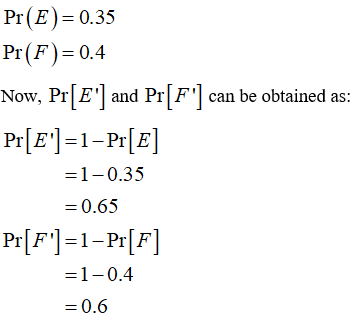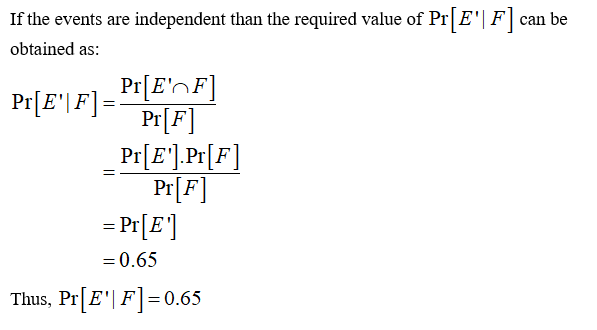# Rework problem 8 from section 3.2 of your text, about independent events E and F in a sample space S, but assume that Pr[E]=0.35 and Pr[F]=0.4.Compute the following conditional probabilities:Pr[E′|F]=Pr[E|F′]=

Question
1 views

Rework problem 8 from section 3.2 of your text, about independent events E and F in a sample space S, but assume that Pr[E]=0.35 and Pr[F]=0.4.

Compute the following conditional probabilities:

Pr[E′|F]=

Pr[E|F′]=

check_circle

Step 1

From the provided information,Step 2...

### Want to see the full answer?

See Solution

#### Want to see this answer and more?

Solutions are written by subject experts who are available 24/7. Questions are typically answered within 1 hour.*

See Solution
*Response times may vary by subject and question.
Tagged in